# JavaScript DOM Nodes

Every element in an HTML page represents a DOM node. These elements are related to each other through the parent-child relationship.

All the nodes in a document make a DOM tree, which describes the relationship.

An element inside another element is known as the child element or child node and the element that contains the element within it is known as the parent element or parent node.

Let's take an example to understand about nodes in JavaScript.

`<P>This is JS DOM</P>`

In the above code, there are two nodes, P and a text node. The combination of both the nodes represents a paragraph. Let's take another code fragment to understand it better.

`<P>This is <B>JS DOM</B></P>`

The above code fragment, created one parent node (P) and two child nodes (text and B node). Further, B node also contains a child node (text).

Following figure shows how the elements of the preceding code fragment can be represented in the form of a tree.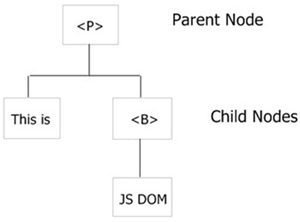## JavaScript DOM Nodes Example

Here are some examples of DOM Nodes in JavaScript.

### Verify Type of Node

Here is an example demonstrates how to check the node type in JavaScript:

```<!DOCTYPE HTML>
<html>
<head>
<title>JavaScript DOM Nodes - Check Node Type</title>
<script type="text/javascript">
function checkNodeType()
{
var tag = document.getElementById("par1");
var value = tag.nodeType;
if(value == 1)
document.write("This is Element Node Type");
if(value == 2)
document.write("This is Attribute Node Type");
if(value == 3)
document.write("This is Text Node Type");
if(value == 8)
document.write("This is Comment Node Type");
if(value == 9)
document.write("This is Document Node Type");
}
</script>
</head>
<body>

<h3>JavaScript DOM Nodes Examples</h3>
<p id="par1">Welcome to JavaScript DOM</p>
<input type="button" onclick="checkNodeType();" value="Check Node Type">

</body>
</html>```

Here is the sample output produced by the above JavaScript DOM Nodes example. This is the initial output: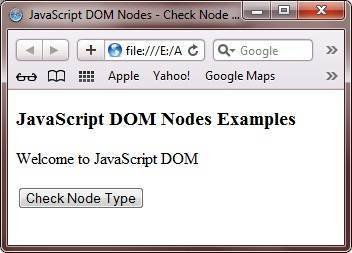Click on the Check Node Type to check as shown in the following figure: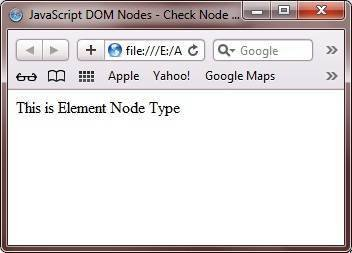### Verify Child Nodes of Node

Here is an example shows how to verify child nodes of node:

```<!DOCTYPE HTML>
<html>
<head>
<title>JavaScript DOM Nodes - Check Child Nodes</title>
<script type="text/javascript">
function checkNode(value)
{
var tag = document.getElementById(value);
if(tag.hasChildNodes())
{
alert("Node has child nodes");
}
else
{
alert("Node has no child nodes");
}
}
</script>
</head>
<body id="T1">

<h3>JavaScript DOM Nodes Examples</h3>
<p>Click on the button given below to check the child nodes of the BODY element.</p>
<input type="button" onclick="checkNode('T1');" value="Check Child Node">
<p>Click on the button given below to check the child nodes of the INPUT element.</p>
<input type="button" id="T2" onclick="checkNode('T2');" value="Check Child Node">

</body>
</html>```

Here is the sample output produced by the above JavaScript DOM Check child nodes example code: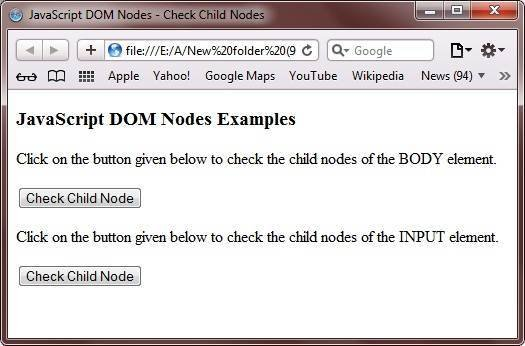Now click on both the Check Child Node button. Here is the output produced on clicking on the first Check Child Node button: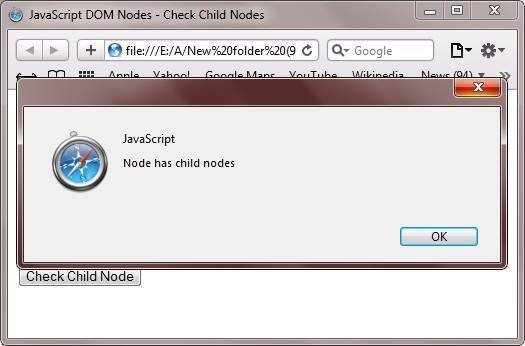Here is the output produced on clicking on the second Check Child Node button: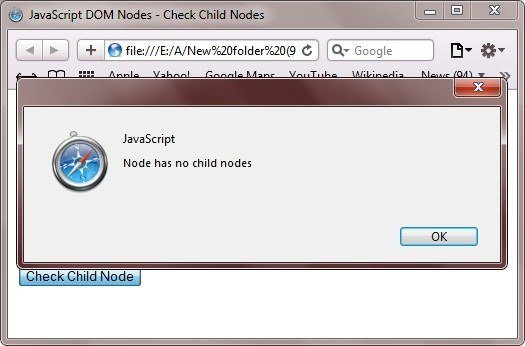Tools
Calculator

Quick Links
Signup - Login - Give Online Test